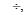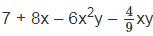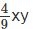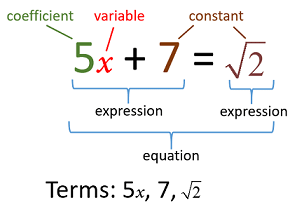Short Notes: Polynomials

# Short Notes: Polynomials - Mathematics (Maths) Class 9

## Polynomial

• An algebraic expression, in which the variables involved have only whole number powers, is called a polynomial.
• Polynomials of degree 1, 2 and 3 are called linear, quadratic and cubic polynomials respectively.
• Polynomials containing one, two and three non-zero terms are called monomial, binomial and trinomial respectively.
• If f(x) be a polynomial of degree n ≥ 1 and let ‘a’ be any real number, then f(a) is the remainder for ‘f(x) being divided by (x – a)’.
• Let f(x) be a polynomial of degree n ≥ 1, then (x – a) is a factor of f(x) provided f(a) = 0. Also if (x + a) is a factor of f(x), then f(–a) = 0

### Algebraic Identities

• x3 + y3 = (x + y)(x2 – xy + y2)
• x3 – y3 = (x – y)(x+ xy + y2)
• x3 + y3 + z3 – 3xyz = (x + y + z)(x2 + y2 + z2 – xy – yz – zx)
• If x + y + z = 0, then x3 + y3 + z= 3xyz
• (x + y + z)2 = x2 + y+ z2 + 2xy + 2yz + 2zx
• (x + y)3 = x3 + y+ 3xy (x + y)
• (x – y)3 = x3 – y3 – 3xy (x – y)

## Variable

A symbol that can be assigned different numerical values is known as a variable. Variables are generally denoted by x, y, z, p, q, r, s, etc.

### Constant

A symbol having a fixed value is called a constant, e.g. 8, 5, 9, p, a, b, c, etc. are constants.

Note: The values of constants remain the same throughout a particular situation, but the value of a variable can keep changing.

## Algebraic Expression

A combination of constants and variables, connected by some or all the basic operations +, –, x,is called an algebraic expression.

Example:is an algebraic expression.

### Terms

Various parts of an algebraic expression separated by (+) or (–) operations are called terms.
Examples: (i) In the above algebraic expression, terms are 7, 8x, –6x2y and(ii) Various terms of 3p4 – 6q2 + 8r3s – 2pq + 6s3 are: 3p4, –6q2, 8r3s, –2pq and 6s3Algebraic Expression

## Polynomial

An algebraic expression in which the variables involved have non-negative integral powers is called a polynomial.

Examples: (i) x3 + x2 – 4x – 7;
(ii) 3p3 + 5p – 9;
(iii) x2 + 2x; etc. are all polynomials

Note: I. Each variable in a polynomial has a whole number as its exponent.

II. Each term of a polynomial has a coefficient.

### Degree of a Polynomial

I. In the case of a polynomial involving one variable, the highest power of the variable is called the degree of the polynomial.
Example: The degree of x5 – 2x3 + x is 5.

II. In the case of a polynomial involving more than one variable, the highest sum of exponents of variables in any term is called the degree of the polynomial.
Example: The degree of p2 – 6p6q + 5p2q3 – 3q4 is 7
(∵ The term –p6q has the sum of exponents of p and q as 6 + 1, i.e. 7)

### Types of Polynomials

A [On the basis of the number of terms]

(i) Monomial: A polynomial containing only one non-zero term is called a monomial.

(ii) Binomial: A polynomial containing two non-zero terms is called a binomial.

(iii) Trinomial: A polynomial containing three non-zero terms is called a trinomial.

Note: I. A monomial containing a constant only is called a constant polynomial.

II. A monomial containing its term as zero only is called a zero polynomial.

III. If we add polynomials, we get a polynomial.

IV. If we multiply polynomials, we get a polynomial.

B [On the basis of its degree]

(i) Linear Polynomial: A polynomial of degree 1 is called a linear polynomial.

(ii) Quadratic Polynomial: A polynomial of degree 2 is called a quadratic polynomial.

(iii) Cubic Polynomial: A polynomial of degree 3 is called a cubic polynomial.

(iv ) Biquadratic Polynomial: A polynomial of degree 4 is called a biquadratic polynomial.

The document Short Notes: Polynomials | Mathematics (Maths) Class 9 is a part of the Class 9 Course Mathematics (Maths) Class 9.
All you need of Class 9 at this link: Class 9

## Mathematics (Maths) Class 9

42 videos|378 docs|65 tests

## FAQs on Short Notes: Polynomials - Mathematics (Maths) Class 9

 1. What is a polynomial?Ans. A polynomial is an algebraic expression consisting of variables and coefficients, combined using addition, subtraction, and multiplication operations. It can have one or more terms, where each term is a combination of a variable raised to a non-negative integer exponent and a coefficient.
 2. What are the different types of polynomials?Ans. There are several types of polynomials based on the number of terms they have. Some common types include: - Monomial: A polynomial with only one term. - Binomial: A polynomial with exactly two terms. - Trinomial: A polynomial with exactly three terms. - Quadratic polynomial: A polynomial of degree 2. - Cubic polynomial: A polynomial of degree 3. - Polynomial of higher degree: A polynomial with more than three terms and degree greater than 3.
 3. How do you add polynomials?Ans. To add polynomials, you simply combine like terms. Like terms are those that have the same variable raised to the same exponent. Add or subtract the coefficients of these like terms while keeping the variable and exponent the same. If a variable does not have a corresponding term in the other polynomial, it remains unchanged in the sum.
 4. What is the degree of a polynomial?Ans. The degree of a polynomial is the highest power of the variable present in the expression. It is determined by looking at the exponent of the variable in each term and selecting the largest one. The degree helps classify polynomials and provides information about their behavior and properties.
 5. Can a polynomial have negative exponents?Ans. No, a polynomial cannot have negative exponents. In a polynomial, the exponents of the variables must be non-negative integers. Negative exponents would result in fractions or decimal values, which do not fit the definition of a polynomial.

## Mathematics (Maths) Class 9

42 videos|378 docs|65 testsExplore Courses for Class 9 examSignup to see your scores go up within 7 days! Learn & Practice with 1000+ FREE Notes, Videos & Tests.
10M+ students study on EduRev
Track your progress, build streaks, highlight & save important lessons and more!
Related Searches

,

,

,

,

,

,

,

,

,

,

,

,

,

,

,

,

,

,

,

,

,

;